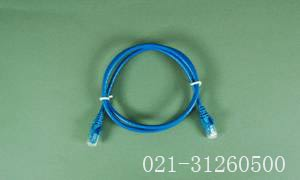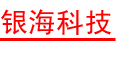## 服务热线 021-31260500

##### 连接件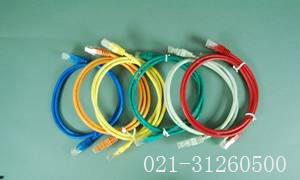#### 超五类非屏蔽RJ45跳线

*：跳线长度，"1"=1米 "2"=2米 "3"=3米 依此类推
#：颜色，A=黑 B=蓝 C=红 D=橙 E=黄 F=灰 G=绿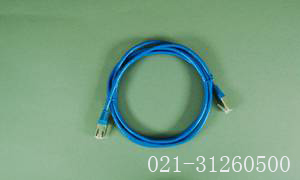#### 超五类屏蔽RJ45跳线

*：跳线长度，"1"=1米 "2"=2米 "3"=3米 依此类推
#：颜色，A=黑 B=蓝 C=红 D=橙 E=黄 F=灰 G=绿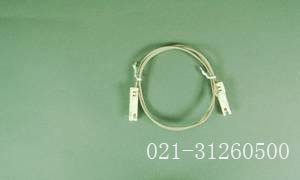#### 1对压接跳线

*：跳线长度，"1"=1米 "2"=2米 "3"=3米 依此类推
#：颜色，A=黑 B=蓝 C=红 D=橙 E=黄 F=灰 G=绿